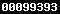### PENGARUH PENERAPAN MODEL PEMBELAJARAN KOOPERATIF TIPE JIGSAW DENGAN TEKNIK PEMBELAJARAN MAKE A MATCH DAN NUMBERED HEADS TOGETHER TERHADAP PRESTASI BELAJAR MATEMATIKA DITINJAU DARI KECERDASAN GANDA SISWA

Nurani Nurani

#### Sari

ABSTRACT

The objectives of this research are to investigate: (1) which learning model of the J-MAM, J-NHT, and conventional learning results in a better learning achievement in Mathematics; (2) which multiple intelligence of the Logical-Mathematical, Visual-Spatial, and Interpersonal types results in a better learning achievement in Mathematics; (3) in each multiple intelligence, which learning model of the J-MAM, J-NHT, and conventional learning results in a better learning achievement in Mathematics; and (4) in each learning model, which multiple intelligence of the Logical-Mathematical, Visual-Spatial, and interpersonal types results in a better learning achievement in Mathematics. This research used the quasi experimental research method with the factorial design of 3 x 3. Its population was all of the students in Grade XI of State Vocational High Schools in Sragen regency. The samples of the research were taken by using the stratified random sampling technique. The data of the research were analyzed by using the unbalanced two-way analysis of variance at the significance level of 5%. The results of the research are as follows: 1) The J-MAM and J-NHT learning models result in the same good learning achievement in Mathematics, but both result in a better learning achievement in Mathematics than the conventional learning model, 2) There are no any differences in the learning achievement in Mathematics of the students with the Logical-Mathematical, Visual-Spatial, and Interpersonal types, 3) In each multiple intelligence type, the J-MAM and J-NHT learning models result in the same good learning achievement in Mathematics, but both result in a better learning achievement in Mathematics than the conventional learning model, 4) In each learning model, the students with Logical-Mathematical, Visual-Spatial, and Interpersonal types have the same learning achievement in Mathematics.

Keywords: Jigsaw learning model, conventional learning model, multiple intelligence, learning achievement in Mathematics.

PDF

### Refbacks

• Saat ini tidak ada refbacks.View My Stats